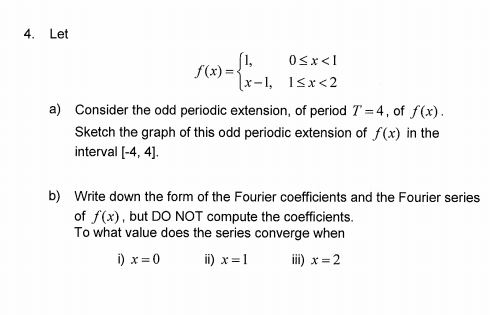Solved: Let 4 0sx

By |Let 4 0sx<I f(x) x-1, 1sx<2 Consider the odd periodic extension, of period T 4, of f(x) Sketch the graph of this odd periodic extension of f(x) in the interval [-4, 4] b) Write down the form of the Fourier coefficients and the Fourier series of f(x), but DO NOT compute the coefficients. To what value does the series converge when i) x 0 ii x 2